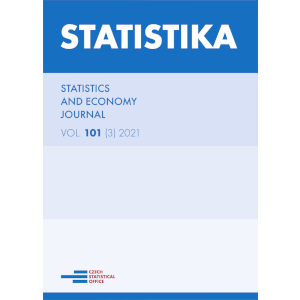Renata Benda-Prokeinová, Martina Hanová, Natalia Turčeková

# Sensitivity Analysis of Price Indices in Models of Demand Systems

Číslo: 3/2021
Periodikum: Statistika

Klíčová slova: Variance, expenditure elasticity, consumer demand model, meat items

Pro získání musíte mít účet v Citace PRO.

Anotace: The primary motivation of the paper is to point out the sensitivity of price indices calculated by the model

of demand systems in different price and quantitative levels. We simulated different prices and quantities,
so increasing the values of the variables reduced their variance. We would like to point out the significant
role of variability and thus the deviation of input data, which is the basis for identifying consumer behavior.
From the methodology, we used the Linearized Almost Ideal Demand System and focused on the partial
output of specific expenditure elasticities calculated by price indices – Stone, Laspeyres and Törnqvist index.
Following we are wondering which index can consider as the most trustworthy?
In the analysis, we realized that the price variance would affect the indices' values more significantly, than
the more considerable variance of the quantity consumed. It means that elasticities characterize consumer
behavior in terms of prices, not in terms of quantity consumed.